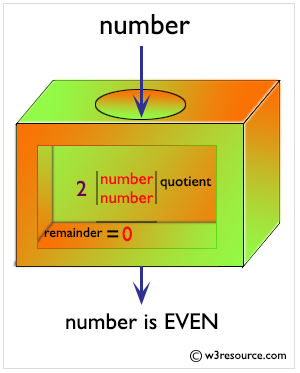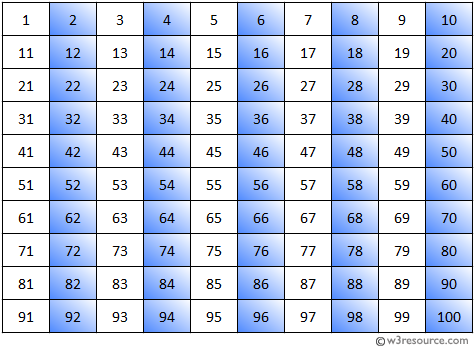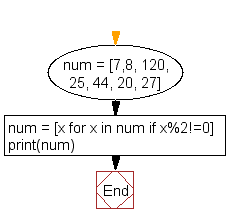﻿ Python: Print the numbers of a specified list after removing even numbers from it - w3resource# Python: Print the numbers of a specified list after removing even numbers from it

## Python List: Exercise - 14 with Solution

Write a Python program to print the numbers of a specified list after removing even numbers from it.

Sample Solution:-

Python Code:

``````num = [7,8, 120, 25, 44, 20, 27]
num = [x for x in num if x%2!=0]
print(num)
```
```

Calculating a Even Numbers:Sample Output:

```[7, 25, 27]
```

Even Numbers between 1 to 100:Flowchart:## Visualize Python code execution:

The following tool visualize what the computer is doing step-by-step as it executes the said program:

Python Code Editor:

Have another way to solve this solution? Contribute your code (and comments) through Disqus.

What is the difficulty level of this exercise?

Test your Python skills with w3resource's quiz

﻿

## Python: Tips of the Day

```print(2_000_000)
```2000000